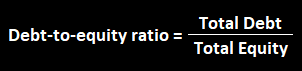# What Is Debt To Equity Ratio?

## Debt To Equity

The debt to equity ratio shows a company’s debt as a percentage of its shareholder’s equity.If the debt to equity ratio is less than 1.0, then the firm is generally less risky than firms whose debt to equity ratio is greater than 1.0.

If the company, for example, has a debt to equity ratio of .50, it means that it uses 50 cents of debt financing for every 1 of equity financing.

View:

The lesser the better as leverage comes with a cost, However, depending on the industry and returns it difference

Where to find the data?

Debt: On the balance sheet liability side

Equity: On the balance sheet equity side